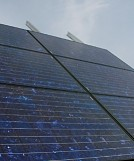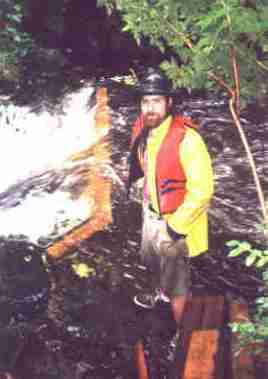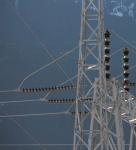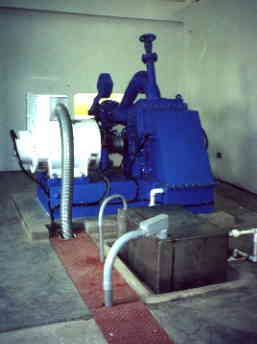Home Power Systems

##### Electricity from the Sun, Wind and Water
DC Hydro Systems
AC Hydro systems
Solar Equipment
Basic Electricity
>Formulas & Tables
Communications
Equipment sales
Contact and FormsOn this page:     Pipe sizing   Units of measure   Fuel costs   Weir tables   Power calculations   Wire tables

Pipe sizing example:

A site has 50 meters of head and a flow of 5 liters per second, a very typical situation here on the coast. The pipeline will have to be 150 meters long, and is going to be polyethylene plastic, (poly pipe). We will accept a head loss of 10% at maximum flow, that is, 5 meters of height lost to pipe friction. The power line length is 50 meters to the battery.
Refer to the section on "Power Calculations" for more detailed explanations of these formula.

Step 1.

Determine the available power.
5 liters per second is 0.005 cubic meters per second )
Power = ( 1000 x Q x h x 9.8 ) x efficiency) watts
Power = ( 1000 x 0.005 x 50 x 9.8 ) x 0.5 ) watts
Power = ( 5 x 50 x 10 ) x 0.5 = 1250 watts

Note: the acceleration due to gravity value of "9.8" was rounded up to "10"
The final output will be somewhat less as the net head will be 45 meters at full flow, or around 1125 watts, 90 % of the last calculation. This is close enough, and we decide to proceed with the project.

Step 2.

Plot a flow of 300 liters per minute on a friction chart applicable to the pipe material you are using, so the flow velocity is less than 5 feet per second. Note the pipe size indicated, and draw lines for several sizes that are available. This will likely be 2 inch, 2.5 inch, 3 inch and 4 inch. Pipe friction charts are available from pipe suppliers. Each type of pipe will have a different coefficient of friction, so a chart used for steel will not be accurate for plastic pipe.

From the chart ...
2 inch gives a velocity of 8.2 feet per second which is too fast.
2.5 inch results in 5.2 feet per second.
3 inch gives 3.7 feet per second.

Pipe inside diameters vary with pipe schedule, (wall thickness), so you will seldom get exactly the diameters listed. Adjust the line you drew on the graph to match the pipe diameter you will be using. This is extremely important if you are using small diameter pipe like 1 inch or 1.25 or 1.5 inch PVC. Some pipe is sold by schedule and some by 'DR' rating (dimension ratio). On long runs of 1000 feet, even the slight differences in inside diameter can make a big difference to head loss. Be careful when making calculations.

 If you really want to know the PVC pipe loss, here is the standard 'Hazen-Williams' formula that is used for pressure pipe friction loss. You can also Google it to find more information on the subject. f= 0.2083 (100/C)^1.852 X Q^1.852 / id^4.865 where f = friction loss per 100 feet C = coefficient of friction, usually 150 for PVC Q = water flow in USGM id = pipe inner diameter For Poly pipe, C is often 140 meaning higher friction. Always try to find out the C for your pipe and the exact id.

Our allowable head loss is 5 meters in 150 meters of pipe length, or 3.33 meters per 100 meters of pipe. Charts are usually normalized to meters per 100 meters or feet per 100 feet, so you may want to convert at this point. In this example, 2.5 inch poly pipe will deliver the required maximum flow with 3.3 meters of head loss per 100 meters of pipe, and the flow velocity is right on 5 feet per second.

It is possible to run more water through the 2.5 inch pipe by increasing the nozzle size. This will increase the head loss beyond our limits, but it will produce more power. In fact we could drop to 70% of the gross head before the power curve would start to fall. However, wide ranges of pressure result in variable jet velocities which in turn determine turbine RPM. In DC systems this is often acceptable, but in AC systems, RPM must be maintained, and a low jet velocity results is a significant loss of efficiency. This is why hydro equipment is so site specific, what worked well at one site may perform badly at another.

Step 3.

Determine the correct nozzle diameter to accept a maximum of 5 liters per second. Often, several nozzles are cut and installed to match flow conditions. 'Stream Emgine' turbines used on some of the example sites featured here are equipped with 2, 3 or 4 nozzles. Each nozzle can have a control valve permitting a wide range of flow to suit conditions. In larger AC systems, spear nozzles are often used which permit continuous adjustments of flow by varying the position of a movable spear within the nozzle.
Refer to a nozzle flow table to determine the correct size to use, or start small and gradually increase the size while measuring the flow in a bucket or through a weir.

Since water is non compressible, the flow velocity and nozzle size can be calculated from scratch. One only needs these formulas. The flow will typically be 5% less than the jet area suggests, so cut it a fraction big. Also, jet velocities are often about 5% less than calculated due to inefficiencies in the nozzle and other complex factors.

Area = 3.1428 x R^2   (Radius)
Circumference = 3.1428 x D   (Diameter)
Jet velocity = 8 x H ^ 1/2 ( 8 times the square root of the net height in feet)

Imagine a solid bar of water passing through the nozzle traveling at the jet velocity. One only needs to determine the bars volume per unit time as it passes by. This is usually in one second. The diameter and hence cross sectional area are found first, then simply multiply this by the 'bars' length passing per second to arrive at the volume. This is easy to do in cubic inches and linear inches, then convert to gallons or litres. See the conversion chart below.

Units of measure:

Any time we deal with the subject of energy, power, pressure or heat, various definitions are required to make meaningful calculations and comparisons. This section explains the meaning of the 'SI' or metric units, and compares them to the imperial or US units.

Every attempt has been made to ensure accuracy on these figures. In all your calculations, be sure the result makes sense, and that you are not getting a result that seems out of proportion to the input numbers. In dealing with energy transfer, be sure to factor in losses due to in-efficiencies. This is very important when comparing costs from the BTU's produced from burning fossil fuels to KWh / BTU's achieved from electrical heat sources.

Definitions
 Ampere -Unit of electrical current, a quantity of electron flow equal to 6 * 1018 electrons / second. Analogous to water volume or quantity flowing in a pipe.
 Volt -Unit of potential difference or electromotive force. Analogous to water pressure in a pipe. In a house, normal wall voltage is 117 volts. Stoves and dryers use 240 volts.
 Watt -Unit of power, the product of amps times volts. The rate of work done in a set unit of time. Equal to the energy spent by one amp flowing through one ohm for one second.
 Kilowatt -Unit of power, one thousand watts
 Kilowatt Hour -Unit of power, the rate of work done in a set unit of time. In this case one hour. If you run a 100 watt bulb for 10 hours, that equals 100 watts times 10 hours = 1000 watt hours, or one kilowatt hour. Electricity is usually bought and sold using block units of kilowatt hours, (1000 watts for a period of time). Normal utility billing is every month or two, so if you use say 15 kilowatts over a 24 hour period for 60 days, that will work out to 15 kW hours a day times 60 days = 900 kw hours. At 7 cents a kilowatt hours, that will cost you \$ 63 dollars. It is important to note that you can use up the electricity at any rate you need during a day, the meter just counts the total used per billing period.
 Ohm -The measure of electrical resistance equal to that resistance which dissipates energy at the rate of one watt from a current of one ampere.

Joules - kilowatts - BTU's
 1 Joule 1 Joule 1 Joule 1 GJ 1 BTU 1 kW 1 kWh 1 kWh 1 watt flowing for one second 1 Newton / metre 0.000948 BTU 948,000 BTU 1054.6 Joule 3412 BTU / hr 3412 BTU 3.6 Mega Joule

Definition of a BTU
Energy required to heat one pound of water by 1 degree F.
10 BTU = 10 pounds (1 imperial gallon ) heated by 1 degree F.

Energy
 1 Watt hour 1 kWh 1 Therm 3.413 BTU 3413 BTU 100000 BTU

Power and Heat flow
 1 kW 1 kW 1 Ton 1 HP 1 hp 0.948 BTU/sec = 3413 BTU/hr 1.3415 HP = 738 ft lb/sec = 44,268 ft lb/min 12000 BTU/hr 0.764 kW = 2546 BTU/hr 0.7455 kW = 550 ft lb/sec = 33000 ft lb/min

Power and Heat flow
1 kW 0.948 BTU/sec = 3413 BTU/hr
1 kW 1.3415 HP = 738 ft lb/sec = 44,268 ft lb/min
1 Ton 12000 BTU/hr
1 hp 0.764 kW = 2546 BTU/hr
1 hp 0.7455 kW = 550 ft lb/sec = 33000 ft lb/min

Volumes and conversions.
 litre cubic ft us gallon imp gallon cubic foot cubic meter = US gal * 0.26442 = litres * 28.313 = litre * 3.785 = litre * 4.5459 = US gallons * 7.49 = 1000 litres

Length
 meters feet km miles = feet * 0.3048 = meters * 3.281 = miles * 1.609 = km * 0.621

Pressure
1 PSI = 6894.76 Newtons / sq. m = 6894.76 Pascal
Absolute Pressure = psia = Gauge pressure (psig) + 14.7

Chemical content of fuels
1 ft^3 of natural gas = 1020 BTU (chemical energy constant)
1 gallon (US) # 2 fuel oil = 140,000 BTU
1 gallon (US) propane = 91,200 BTU

Calorie (c)
Heat required to raise 1 gm. water by 1 degree C.
Kilocalorie (C) = 1000 calories. C = food calorie

 Some practical fuel cost examples: Updated Dec 2015. Natural gas is sold by its heating value in 'Giga Joules', not by its volume because the heating ability per unit volume can vary. Since it is a commodity, its price can vary. Electricity is sold by the 'kilowatt hour'. In BC, the level one tier cost for 1 kwh is currently \$0.08, December 2015 price, and \$ 0.12 cents for the level two tier. That is 8.0 cents for 1,000 watts for one hour - or 100 watts for ten hours. So to run a typical 1500 watt electric heater for one hour it costs (1.5kW X 8.0 cents, or about 12 cents an hour) Some definitions you have to understand for this to make sense: ' / ' means per or divided by. * or X mean to multiply. 1 Joule = 1 watt for 1 second (a tiny amount of energy ) 1 Giga Joule = 1 billion Joules (a fixed amount of energy used for billing purposes) For the purpose of these examples, the cost of electricity is 8.0 cents per kW hour. This is ignoring the two tier price system since a careful consumer can keep the monthly use with in the first price tier. This would not apply to a home heated by electricity. 1 Giga Joule is the same amount of energy as 277 kilowatt hours. That is about the amount of electricity used in 15 days in an average home that is heated by natural gas. For an electrically heated home, this figure will be considerably higher in the heating season. In BC, the Dec 2015 cost of 277 kW hours of electricity (1 GJ worth) = 277 X 8.0 cents/kwh = \$ 22.16, taxes extra. (1,376 KWh is the price tier change) The cost of 1 GJ of natural gas (277 kW worth) in Dec 2015 hovers about \$ 3.56 per GJ, but with storage, delivery and PST, the price is \$11.00 per GJ. So you can see that heating with natural gas is presently about half the cost as heating with electricity. Facts about Oil: The New York spot price of one US gallon of heating oil at the end of Dec 2015 is US \$ 01.08, or US \$0.29 cents a liter. Oil, like natural gas, is traded as a commodity on the stock markets and with oil at an all time low in 2015, this price per gallon is much lower than in the past years. Since this price is so volitile of late, take what you read below with some doubt! It is likely a liter of oil would sell for Cdn 90 cents at this time, 2015 price. A typical home oil tank holds 300 US gallons, or 1130 liters. The heating value of 1 liter of # 2 heating oil is 37,000 BTU, or British Thermal Units. (An old, but useful measuring system - based on 140,000 BTU per USA gallon) At 37,000 BTU per liter / 3,413 BTU per kW hour = 10.8 kW hour per liter of heating oil. This means that there is the same amount of energy in one liter of oil as that used by a 1000 watt electric heater running for 10.8 hours. 10.8 kW of electricity at \$ 0.08 per kW = (10.8 X 8.0) = 86 cents And as stated above, 1 liter of heating oil costs about 90 cents. Add to that the lower efficiency of some gas or oil furnaces and the real cost is somewhat more. If the furnace is 75 % efficient, then 25 % of the cost is going to waste. Electric heat on the other hand, although also quite expensive, is nearly 100 % efficient, so all the power used is turned to heat at the electric heater. If however you heated with electricity, then with tier two at 12 cents per KW, electricity would likely be more expensive.
Weir tables:

Weir tables provide a quick and accurate means to determine water flow by measuring water depth flowing through a calibrated
notch. The following table shows depth in inches and flow in cubic feet per second and in liters per second through a 90 degree, sharp edged V notch.

90 Degree V notch weir table.
 Depth / inch 4 5 6 7 8 9 10 11 12 13 14 15 16 Flow / cfs 0.16 0.28 0.44 0.65 0.91 1.22 1.58 2.01 2.5 3.05 3.67 4.37 5.13 Flow / l/s 4.53 7.93 12.4 18.4 25.8 34.5 44.7 56.9 70.8 86.3 104 124 145

Four foot wide horrizontal notch weir table.
 Depth / inch 1 2 3 4 5 6 7 8 9 10 11 12 13 Flow / cfs 0.3 0.9 1.6 2.5 3.5 4.6 5.7 7 8.3 9.7 Flow / l/s 8.5 25.5 45.3 70.8 99.1 130 161 198 235 275

 Power Calculations : In SI units, (metric) The energy available in falling water is expressed as, E = mgh   where... e = energy = ( m g h ) Joules (a Joule is one watt for one second) m = mass, the product of density (p) x volume (V) g = gravitational constant of acceleration, 9.8 meters per second per second h = head in meters Note: time is not a factor in this relationship for energy. Power is defined as energy released over a specific time, so substituting in the values above,      Power = ( p V g h ) Joules / second (same as watts) The density of water is 1000 kg/m^3, (or 1 kg/liter), and the gravitational constant of acceleration on earth is 9.8 m/s^2, (the force that gives 'mass' its weight). In hydro applications, the letter 'Q' is used to represent the volume of water combined with its density. Substituting in gives gross power equal to, Power = ( 1000 x Q x 9.8 x h ) watts,  or in kW Power = (1000 x Q x 9.8 x h ) / 1000 kilowatts, and futher reduced to...     Power = (Q x 9.8 x h ) kilowatts,   ( with Q in cubic meters per second ) Note: as mentioned above, see how the "V" for volume has been replaced by "Q" for quantity representing 1 cubic meter of water. Note: See how this is similar to the energy equation above, energy = m(Q) x g(9.8) X h Overall system efficiency is often around 55 - 65 % in systems under a kW, so you can round off the above to the following for rough working. Power = ( Q x h x 10 x 0.6 ) kW ... for small systems where Q is volume in cubic meters per second, and h is head in meters. For plants in the multi kW range, efficiencies reach 70 percent or higher. Actual efficiency depends on the water flow characteristics, nozzle design, turbine design, coupling, and generator. Power line losses, battery charge / discharge losses and inverter effiency must also be considered. Example: You have a stream with a flow of 3 liters per second and a drop of 50 meters available. What is the gross power potential in watts ? 3 liters per second is 0.003 cubic meters per second) Power = ( 1000 x Q x h x 9.8 x efficiency) watts Power = ( 1000 x 0.003 x 50 x 10 ) watts Now multiply this result by the expected overall efficiency. (say 60%) Power = ( 3 x 50 x 10 ) x 0.6 = 900 watts (rounded off) In Imperial units: Total energy available is expressed as, energy = Q x 62.4 x h Q = cubic feet of water per minute 62.4 = density of water, @ 62.4 lbs. per cubic foot h = net head in feet This is really foot pounds of potential energy per minute, (so many pounds at so many feet head.) One horsepower is equivalent to 33,000 foot pounds of work done in 1 minute, so to figure in HP, Q X 62.4 X h / 33,000 or Q x h / 529 Since 1 Hp is equivalent to 745 electrical watts, convert to kW by Power in kW =(Q x 62.4 x h / 33,000 ) X 0.745 or, Power in kW = (Q x H / 709) where Q is in cubic feet per minute. In all cases it is important to multiply the gross power result by the system efficiency, again use 55- 65 % for small systems, and perhaps 70 % for larger system. On larger sites, it is often easier to think of cubic feet in one second, so convert the above to... Power in kW = ( Q x h / 11.8 ) where Q is now in cubic feet per second. For general work on small systems, the following formula is easy to remember and works well. It assumes an overall efficiency of 55 %, so the result is close to the power you will get. Power in watts = (net head in feet x flow in USGM) / 10   (again, at an overall efficiency of 55 %)

Wire Tables:

This is a standard AWG wire table. Values have been checked against several reliable sources.

* Ampacity listed is for 90 degree wires or cables in bundles or conduit. Check with your local regulations on specific allowable ampacity or other considerations. *

The intended use of aluminum wire here is only for low voltage, 12 to 48 volt applications where it is essential to keep the total voltage drop and corresponding power loss to a minimum. A number 2/0 aluminum conductor has a resistance of only 0.128 ohms per 1000 feet, comparable to a number 1 copper conductor, but the cost for aluminum is much less than for copper of a similar resistance. Large diameter single conductor aluminum wires must be placed in conduit and buried. Aluminum wire is much softer than copper and it must be terminated appropriately to prevent corrosion.

Rather than give the one or two way distances you can run a particular power level, it is better to calculate the voltage drop based on wire resistance and ampacity to convay. The method is described just below this table.

 Wire Guage Ohms / 1000 ft. Cu Ampacity (see note *) Ohms / 1000 ft. Al Ampacity (see note *) 0000 .050 235 0.0804 # 000 .063 210 0.101 # 00 .077 185 0.128 # 0 .096 155 0.161 # 1 .126 140 0.203 # 2 .159 120 0.256 # 3 .200 105 0.323 # 4 .253 85 0.408 # 6 .403 65 0.648 # 8 .640 45 1.03 # 10 1.018 30 1.64 # 12 1.619 20 2.61 # 14 2.575 15 4.14 # 16 4.094 8 6.59 #

 For a DC system, or single phase AC, there will be two wires carrying current, positive and negative, or hot and neutral for AC. Calculate the total resistance of both wire runs, (there and back). Values given are for 1000 feet, so use the ratio of your distance to 1000 feet * the resistance indicated per 1000 feet. Example: for 350 feet from the generator to the battery, it would be 700 feet total wire. Say you are using # 6 which has a resistance of 0.403 ohms per 1000 feet. Your resistance would be 700 / 1000 * 0.403 = 0.282 ohms Determine the voltage drop by multiplying the resistance you come up with by the anticipated current, (amps in the wire). (Amps = power in watts / system voltage) This voltage will subtract from the generator voltage, so in the above example, if the generator is producing some DC voltage, and we expect 20 amps at 26 volts at the load, that means there is 20 amps flowing through the 0.282 ohms of wire which causes a voltage drop of ( 0.282 * 20 = 5.64 volts drop) Depending on the generator, it will either spin faster and produce a higher voltage to overcome this loss, and still provide the 20 amps at the load, or it may get maxed out unable to produce more voltage, and the voltage drop will limit the ability to push the current to the load. (Note, voltage is like pressure which pushes the Amps. Amps are like the volume of electricity in the wire, hence the name 'current').
Top